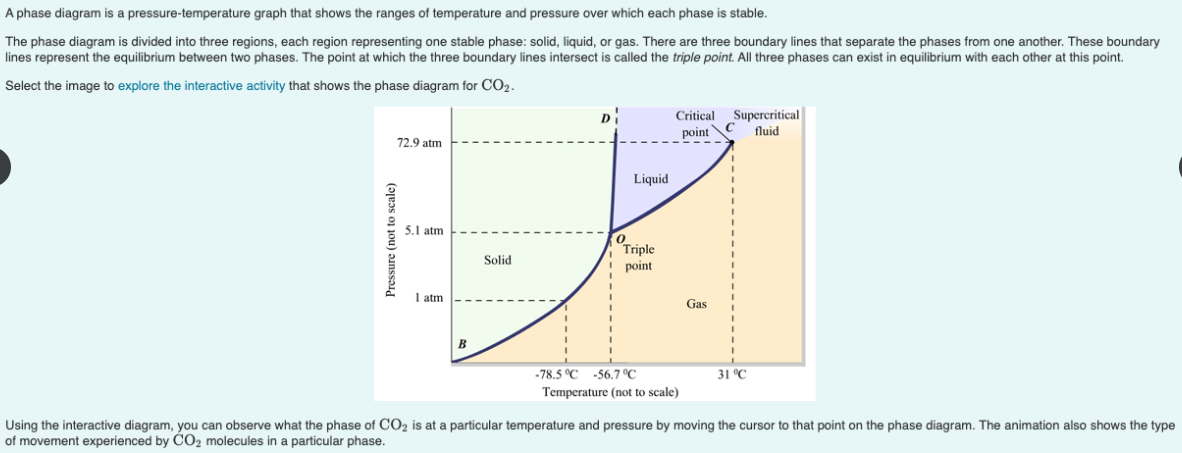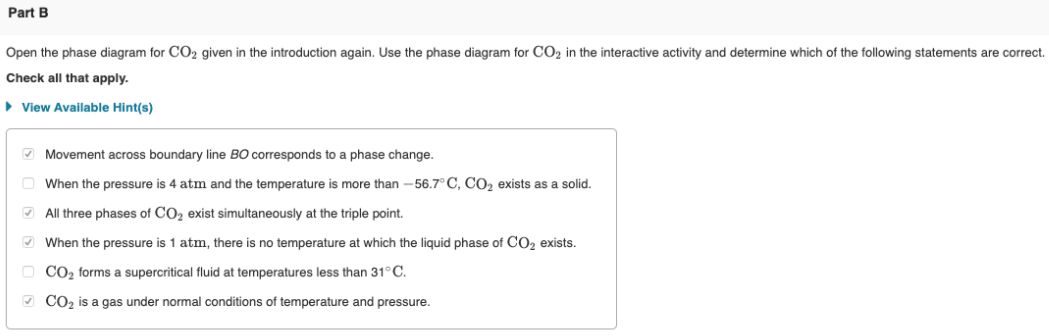# A phase diagram is a pressure-temperature graph that shows the rangers of temperature and pressure over which each phase is stable. The phase diagram is divided into three regions, each region representing one stable phase: solid, liquid, or gas. There are three boundary lines that separate the phases from one another. These boundary lines represent the equilibrium between two phases. the point at which the three boundary lines intersect is called the triple point. All three phases can exist in equilibrium with each other at this point. Select the image to explore the interactive activity that shows the phase diagram for CO2. Using the interactive diagram, you can observe what the phase of CO2 is at a particular temperature and pressure by moving the cursor to that point on the phase diagram. The animation also shows the type of movement experienced by CO2 molecules in a particular phase. Open the phase diagram for CO2 given in the introduction again. Use the phase diagram for CO2 in the interactive activity and determine which of the following statements are correct. Check all that apply.